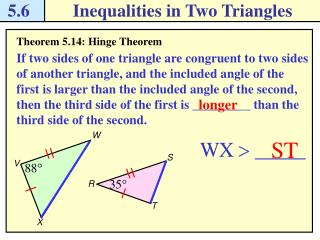# 5.6 - PowerPoint PPT PresentationDownload Presentation5.6

5.6Download Presentation## 5.6

- - - - - - - - - - - - - - - - - - - - - - - - - - - E N D - - - - - - - - - - - - - - - - - - - - - - - - - - -
##### Presentation Transcript

1. 5.6 Inequalities in Two Triangles W V S X R T Theorem 5.14: Hinge Theorem If two sides of one triangle are congruent to two sides of another triangle, and the included angle of the first is larger than the included angle of the second, then the third side of the first is _________ than the third side of the second. longer

2. 5.6 Inequalities in Two Triangles D A B E F C Theorem 5.15: Converse of the Hinge Theorem If two sides of one triangle are congruent to two sides of another triangle, and the third side of the first is longer than the third side of the second, then the included angle of the first is _________ than the included angle of the second. larger

3. 5.6 Inequalities in Two Triangles D B A B E A D C C F Use the Hinge Theorem and its converse Example 1 Complete the statement with <, >, or =. Explain. Solution a. You are given that Because 61o < ____, by the Hinge Theorem, AC < ____.

4. 5.6 Inequalities in Two Triangles D B A B E A D C C F Use the Hinge Theorem and its converse Example 1 Complete the statement with <, >, or =. Explain. Solution b. You are given that and you know that by the Reflexive Property. Because 34 > 33, ____ > ____. So, by the Converse of the Hinge Theorem, ________ > ________.

5. 5.6 Inequalities in Two Triangles Solve a multi-step problem Example 2 Travel Car A leaves a mall, heads due north for 5 mi and then turns due west for 3 mi. Car B leaves the same mall, heads due south for 5 mi and then turns 80o towards east for 3 mi. Which car is farther from the mall? A Draw a diagram. The distance driven and the distance back to the mall form two triangles, with _________ 5 mile sides and __________ 3 mile sides. congruent Mall congruent Add the third side to the triangle. Use linear pairs to find the included angles of _____ and _____. B Because 100o > 90o, Car ___ is farther from the mall than Car A by the _______________. Hinge Theorem

6. 5.6 Inequalities in Two Triangles Write an indirect proof Example 3 Write an indirect proof to show that an odd number is not divisible by 6. Given x is an odd number. Prove x is not divisible by 6. Solution x is divisible by 6 Assume temporarily that ______________________. Step 1 This means that ____ = n for some whole number n. x 6n So, multiplying both sides by 6 gives ___ = ___.

7. 5.6 Inequalities in Two Triangles Write an indirect proof Example 3 Write an indirect proof to show that an odd number is not divisible by 6. Given x is an odd number. Prove x is not divisible by 6. Solution If x is odd, then by definition, x cannot be divided evenly by ___. However, __ = ___ so ___ = ____ = ___. Step 2 3n x 2 6n We know that __ is a whole number because n is a whole number, so x can be divided evenly by __. 3n 2 x is odd This contradicts the given statement that _________. Therefore, the assumption that x is divisible by 6 is _____, false Step 3 which proves that _______________________. x is not divisible by 6

8. 5.6 Inequalities in Two Triangles B A C D Checkpoint. Complete the following exercises. which is longer, AB or CB?

9. 5.6 Inequalities in Two Triangles A Mall B Checkpoint. Complete the following exercises. In example 2, car C leaves the mall, and goes 5 miles due west then turns 85o towards south for 3 mi. Write the cars in order from the car closest to the mall to the car farthest from the mall. Car A is closest Car C is next closest Car B is farthest C

10. 5.6 Inequalities in Two Triangles Checkpoint. Complete the following exercises. • Suppose you want to prove the statement “If x + y = 5 and y = 2, then x = 3.” What temporary assumption could you make to prove the conclusion indirectly? You can temporarily assume that

11. 5.6 Inequalities in Two Triangles Pg. 305, 5.6 #1-15